International
Tables for
Crystallography
Volume D
Physical properties of crystals
Edited by A. Authier

International Tables for Crystallography (2006). Vol. D, ch. 1.1, pp. 3-5

## Section 1.1.1. The matrix of physical properties

A. Authiera*

aInstitut de Minéralogie et de la Physique des Milieux Condensés, Bâtiment 7, 140 rue de Lourmel, 75015 Paris, France
Correspondence e-mail: aauthier@wanadoo.fr

### 1.1.1. The matrix of physical properties

| top | pdf |

#### 1.1.1.1. Notion of extensive and intensive quantities

| top | pdf |

Physical laws express in general the response of a medium to a certain influence. Most physical properties may therefore be defined by a relation coupling two or more measurable quantities. For instance, the specific heat characterizes the relation between a variation of temperature and a variation of entropy at a given temperature in a given medium, the dielectric susceptibility the relation between electric field and electric polarization, the elastic constants the relation between an applied stress and the resulting strain etc. These relations are between quantities of the same nature: thermal, electrical and mechanical, respectively. But there are also cross effects, for instance:

 (a) thermal expansion and piezocalorific effect: mechanical reaction to a thermal impetus or the reverse; (b) pyroelectricity and electrocalorific effect: electrical response to a thermal impetus or the reverse; (c) piezoelectricity and electrostriction: electric response to a mechanical impetus; (d) piezomagnetism and magnetostriction: magnetic response to a mechanical impetus; (e) photoelasticity: birefringence produced by stress; (f) acousto-optic effect: birefringence produced by an acoustic wave; (g) electro-optic effect: birefringence produced by an electric field; (h) magneto-optic effect: appearance of a rotatory polarization under the influence of a magnetic field.

The physical quantities that are involved in these relations can be divided into two categories:

• (i) extensive quantities, which are proportional to the volume of matter or to the mass, that is to the number of molecules in the medium, for instance entropy, energy, quantity of electricity etc. One uses frequently specific extensive parameters, which are given per unit mass or per unit volume, such as the specific mass, the electric polarization (dipole moment per unit volume) etc.

• (ii) intensive parameters, quantities whose product with an extensive quantity is homogeneous to an energy. For instance, volume is an extensive quantity; the energy stored by a gas undergoing a change of volume dV under pressure p is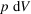. Pressure is therefore the intensive parameter associated with volume. Table 1.1.1.1gives examples of extensive quantities and of the related intensive parameters.

 Table 1.1.1.1| top | pdf | Extensive quantities and associated intensive parameters
 The last four lines of the table refer to properties that are time dependent.
Extensive quantitiesIntensive parameters
Volume Pressure
Strain Stress
Displacement Force
Entropy Temperature
Quantity of electricity Electric potential
Electric polarization Electric field
Electric displacement Electric field
Magnetization Magnetic field
Magnetic induction Magnetic field
Reaction rate Chemical potential
Heat flow Temperature gradient
Diffusion of matter Concentration gradient
Electric current Potential gradient

#### 1.1.1.2. Notion of tensor in physics

| top | pdf |

Each of the quantities mentioned in the preceding sectionis represented by a mathematical expression. Some are direction independent and are represented by scalars: specific mass, specific heat, volume, pressure, entropy, temperature, quantity of electricity, electric potential. Others are direction dependent and are represented by vectors: force, electric field, electric displacement, the gradient of a scalar quantity. Still others cannot be represented by scalars or vectors and are represented by more complicated mathematical expressions. Magnetic quantities are represented by axial vectors (or pseudovectors), which are a particular kind of tensor (see Section 1.1.4.5.3). A few examples will show the necessity of using tensors in physics and Section 1.1.3will present elementary mathematical properties of tensors.

 (i) Thermal expansion. In an isotropic medium, thermal expansion is represented by a single number, a scalar, but this is not the case in an anisotropic medium: a sphere cut in an anisotropic medium becomes an ellipsoid when the temperature is varied and thermal expansion can no longer be represented by a single number. It is actually represented by a tensor of rank 2. (ii) Dielectric constant. In an isotropic medium of a perfect dielectric we can write, in SI units,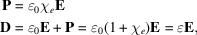where P is the electric polarization (= dipole moment per unit volume),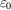the permittivity of vacuum,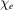the dielectric susceptibility, D the electric displacement andthe dielectric constant, also called dielectric permittivity. These expressions indicate that the electric field, on the one hand, and polarization and displacement, on the other hand, are linearly related. In the general case of an anisotropic medium, this is no longer true and one must write expressions indicating that the components of the displacement are linearly related to the components of the field: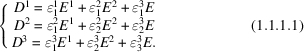The dielectric constant is now characterized by a set of nine components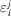; they are the components of a tensor of rank 2. It will be seen in Section 1.1.4.5.2.1that this tensor is symmetric (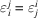) and that the number of independent components is equal to six. (iii) Stressed rod (Hooke's law). If one pulls a rod of length ℓ and cross sectionwith a force F, its length is increased by a quantity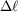given by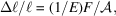where E is Young's modulus, or elastic stiffness (see Section 1.3.3.1). But, at the same time, the radius, r, decreases by Δr given by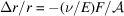, where ν is Poisson's ratio (Section 1.3.3.4.3). It can be seen that a scalar is not sufficient to describe the elastic deformation of a material, even if it is isotropic. The number of independent components depends on the symmetry of the medium and it will be seen that they are the components of a tensor of rank 4. It was precisely to describe the properties of elasticity by a mathematical expression that the notion of a tensor was introduced in physics by W. Voigt in the 19th century (Voigt, 1910) and by L. Brillouin in the first half of the 20th century (Brillouin, 1949). (iv) Expansion in Taylor series of a field of vectors. Let us consider a field of vectors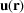where r is a position vector. The Taylor expansion of its components is given by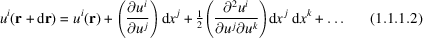using the so-called Einstein convention, which implies that there is automatically a summation each time the same index appears twice, once as a superscript and once as a subscript. This index is called a dummy index. It will be shown in Section 1.1.3.8that the nine partial differentials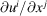and the 27 partial differentials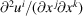are the components of tensors of rank 2 and 3, respectively.

Remark. Of the four examples given above, the first three (thermal expansion, dielectric constant, stressed rod) are related to physical property tensors (also called material tensors), which are characteristic of the medium and whose components have the same value everywhere in the medium if the latter is homogeneous, while the fourth one (expansion in Taylor series of a field of vectors) is related to a field tensor whose components vary at every point of the medium. This is the case, for instance, for the strain and for the stress tensors (see Sections 1.3.1and 1.3.2).

#### 1.1.1.3. The matrix of physical properties

| top | pdf |

Each extensive parameter is in principle a function of all the intensive parameters. For a variation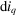of a particular intensive parameter, there will be a variation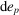of every extensive parameter. One may therefore write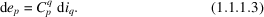The summation is over all the intensive parameters that have varied.

One may use a matrix notation to write the equations relating the variations of each extensive parameter to the variations of all the intensive parameters: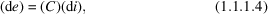where the intensive and extensive parameters are arranged in column matrices, (di) and (de), respectively. In a similar way, one could write the relations between intensive and extensive parameters as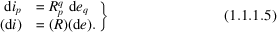Matrices (C) and (R) are inverse matrices. Their leading diagonal terms relate an extensive parameter and the associated intensive parameter (their product has the dimensions of energy), e.g. the elastic constants, the dielectric constant, the specific heat etc. The corresponding physical properties are called principal properties. If one only of the intensive parameters,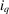, varies, a variationof this parameter is the cause of which the effect is a variation,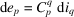(without summation), of each of the extensive parameters. The matrix coefficients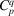may therefore be considered as partial differentials: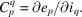The parametersthat relate causesand effectsrepresent physical properties and matrix (C) is called the matrix of physical properties. Let us consider the following intensive parameters: T stress, E electric field, H magnetic field, Θ temperature and the associated extensive parameters: S strain, P electric polarization, B magnetic induction, σ entropy, respectively. Matrix equation (1.1.1.4)may then be written: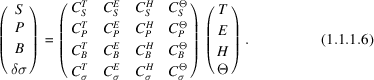The various intensive and extensive parameters are represented by scalars, vectors or tensors of higher rank, and each has several components. The terms of matrix (C) are therefore actually submatrices containing all the coefficientsrelating all the components of a given extensive parameter to the components of an intensive parameter. The leading diagonal terms,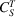,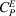,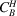,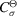, correspond to the principal physical properties, which are elasticity, dielectric susceptibility, magnetic susceptibility and specific heat, respectively. The non-diagonal terms are also associated with physical properties, but they relate intensive and extensive parameters whose products do not have the dimension of energy. They may be coupled in pairs symmetrically with respect to the main diagonal: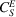and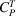represent the piezoelectric effect and the converse piezoelectric effect, respectively;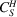and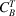the piezomagnetic effect and the converse piezomagnetic effect;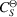and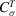thermal expansion and the piezocalorific effect;and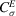the pyroelectric and the electrocalorific effects;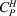and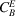the magnetoelectric effect and the converse magnetoelectric effect;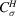and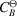the pyromagnetic effect and the magnetocalorific effect.

It is important to note that equation (1.1.1.6)is of a thermodynamic nature and simply provides a general framework. It indicates the possibility for a given physical property to exist, but in no way states that a given material will exhibit it. Curie laws, which will be described in Section 1.1.4.2, show for instance that certain properties such as pyroelectricity or piezoelectricity may only appear in crystals that belong to certain point groups.

#### 1.1.1.4. Symmetry of the matrix of physical properties

| top | pdf |

If parameter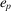varies by, the specific energy varies by du, which is equal to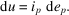We have, therefore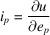and, using (1.1.1.5),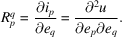Since the energy is a state variable with a perfect differential, one can interchange the order of the differentiations:Since p and q are dummy indices, they may be exchanged and the last term of this equation is equal to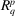. It follows that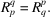Matrices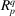andare therefore symmetric. We may draw two important conclusions from this result:

 (i) The submatrices associated with the principal properties are symmetric with respect to interchange of the indices related to the causes and to the effects: these properties are represented by symmetric tensors. For instance, the dielectric constant and the elastic constants are represented by symmetric tensors of rank 2 and 4, respectively (see Section 1.1.3.4). (ii) The submatrices associated with terms that are symmetric with respect to the main diagonal of matrices (C) and (R) and that represent cross effects are transpose to one another. For instance, matrix () representing the converse piezoelectric effect is the transpose of matrix () representing the piezoelectric effect. It will be shown in Section 1.1.3.4that they are the components of tensors of rank 3.

#### 1.1.1.5. Onsager relations

| top | pdf |

Let us now consider systems that are in steady state and not in thermodynamic equilibrium. The intensive and extensive parameters are time dependent and relation (1.1.1.3)can be written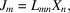where the intensive parameters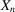are, for instance, a temperature gradient, a concentration gradient, a gradient of electric potential. The corresponding extensive parameters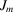are the heat flow, the diffusion of matter and the current density. The diagonal terms of matrix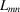correspond to thermal conductivity (Fourier's law), diffusion coefficients (Fick's law) and electric conductivity (Ohm's law), respectively. Non-diagonal terms correspond to cross effects such as the thermoelectric effect, thermal diffusion etc. All the properties corresponding to these examples are represented by tensors of rank 2. The case of second-rank axial tensors where the symmetrical part of the tensors changes sign on time reversal was discussed by Zheludev (1986).

The Onsager reciprocity relations (Onsager, 1931a,b)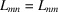express the symmetry of matrix. They are justified by considerations of statistical thermodynamics and are not as obvious as those expressing the symmetry of matrix (). For instance, the symmetry of the tensor of rank 2 representing thermal conductivity is associated with the fact that a circulating flow is undetectable.

Transport properties are described in Chapter 1.8of this volume.

### References

Brillouin, L. (1949). Les tenseurs en mécanique et en élasticité. Paris: Masson & Cie.
Onsager, L. (1931a). Reciprocal relations in irreversible processes. I. Phys. Rev. 37, 405–426.
Onsager, L. (1931b). Reciprocal relations in irreversible processes. II. Phys. Rev. 38, 2265–2279.
Voigt, W. (1910). Lehrbuch der Kristallphysik. Leipzig: Teubner. 2nd ed. (1929); photorep. (1966). New York: Johnson Reprint Corp. and Leipzig: Teubner.
Zheludev, I. S. (1986). Space and time inversion in physical crystallography. Acta Cryst. A42, 122–127.